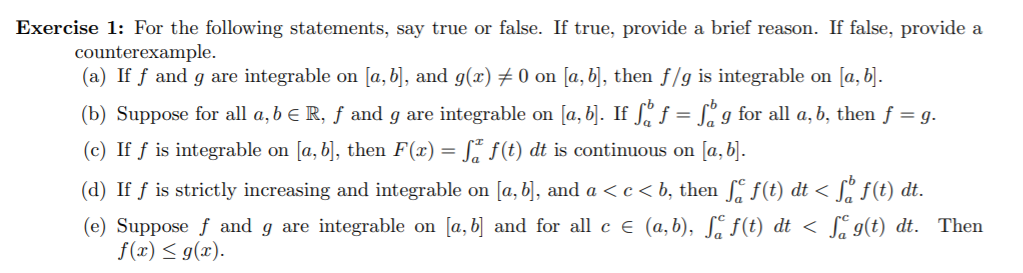# Exercise 1: For the following statements, say true or false. If true, provide a brief reason. If false, provide acounterexample.(a) If f and g are integrable on [a, b], and g(x) # 0 on [a, b], then f/g is integrable on [a, b].(b) Suppose for all a, b e R, ƒ and g are integrable on [a, b]. If Sº f = L° g for all a, b, then f = g.(c) If f is integrable on [a, b], then F(x) = S* f(t) dt is continuous on [a, b].(d) If ƒ is strictly increasing and integrable on [a, b], and a < c < b, then , f(t) dt < [, f(t) dt.(e) Suppose f and g are integrable on [a, b] and for all c e (a,b), S. f(t) dt < S g(t) dt. Thenf(x) < g(x).

Question
19 views

d and ehelp_outlineImage TranscriptioncloseExercise 1: For the following statements, say true or false. If true, provide a brief reason. If false, provide a counterexample. (a) If f and g are integrable on [a, b], and g(x) # 0 on [a, b], then f/g is integrable on [a, b]. (b) Suppose for all a, b e R, ƒ and g are integrable on [a, b]. If Sº f = L° g for all a, b, then f = g. (c) If f is integrable on [a, b], then F(x) = S* f(t) dt is continuous on [a, b]. (d) If ƒ is strictly increasing and integrable on [a, b], and a < c < b, then , f(t) dt < [, f(t) dt. (e) Suppose f and g are integrable on [a, b] and for all c e (a,b), S. f(t) dt < S g(t) dt. Then f(x) < g(x). fullscreen
check_circle

Step 1

Hello. Since your question has multiple sub-parts and you have asked the solution for the subpart d and e only, so we will solve d and e only.

Consider the solution for subpart (d).

The statement given in subpart (d) is True.

It is given that function f is strictly increasing in [a, b] and a < c < b.

Consider the first case that function f and g are strictly increasing in [a, b].

Therefore, f (b) > f (a).

Now, consider the second case that the value if c lies as a < c < b.

Again, f (a) < f (c) < f (b).

Definite integrals show the area under the curve.

Step 2

Consider the solution for subpart (e).

The statement given in subpart (e) is True.

It is given that function f and g are integrable funct...

### Want to see the full answer?

See Solution

#### Want to see this answer and more?

Solutions are written by subject experts who are available 24/7. Questions are typically answered within 1 hour.*

See Solution
*Response times may vary by subject and question.
Tagged in

### Calculus